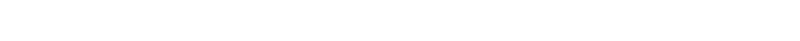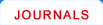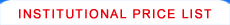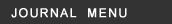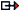Journal HomeEditorial BoardGuidelines for AuthorsSubscriptionContentOnline Submission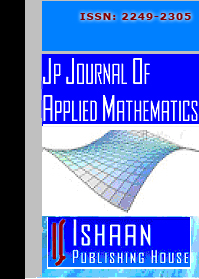JP Journal of Applied Mathematics
Editorial Board
 Ananthaswamy, V. Department of Mathematics The Madura College (Autonomous) Madurai, Tamil Nadu India [Applied differential equations, Applied linear and non linear partial differential equations, Mathematical modeling, Approximation techniques, Perturbation technique, Numerical and mathematical analysis, Computational mathematics, Computational and mathematical modeling, Mathematical biology, Solving nonlinear differential equations in ecological systems] Bermejo, Rodolfo Department of Applied Mathematics ETSII Universidad Politécnica de Madrid José Gutiérrez Abascal 2, 28006 Madrid Spain Boniello, Carmine University of Salerno Fisciano, Salerno Italy [Nash equilibrium, differential equations, marketing, risk theory] Chen, Chi-Ming Department of Applied Mathematics National Hsinchu University of Education Taiwan ROC [Functional analysis, Fixed point theory] Chen, Hongkui Department of Applied Mathematics Chang'an University Xi’an 710064 P. R. China Chen, Yuqing Faculty of Applied Mathematics Guangdong University of Technology Guangzhou, Guangdong 510006 P. R. China [Nonlinear operator theory, Topological degree theory, Variational inequality theory and nonlinear evolution equations] Fard, Omid Solaymani Department of Applied Mathematics School of Applied Mathematics and Computer Science Damghan University Iran [Numerical methods for ordinary, Fuzzy and stochastic differential equations] Haque, Mainul School of Mathematical Sciences University of Nottingham Nottingham, NG7 2RD U. K. [Dynamical systems, Mathematical biology: gene regulatory networks, Mathematical ecology, Epidemiology, eco-epidemiology, Diffusion and pattern formation in medicine and biology, Chaos] Huang, Shuechin Department of Applied Mathematics Dong Hwa University Hualien 97401 Taiwan [Fixed-point theorems" [MSC: 47H10] is my specialty] Huo, Hai-Feng Institute of Applied Mathematics Lanzhou University of Technology Lanzhou, Gansu 730050 P. R. China [Differential equation, Mathbiology] Li, Xiaodi School of Mathematical Sciences Shandong Normal University Ji'nan, 250014, Shandong P. R. China [stability theory, equations with impulses, global stability, neural networks, Lyapunov stability] Merlone, Ugo Department of Statistics and Applied Mathematics University of Torino Corso Unione Sovietica 218 bis, Torino I-10134 Italy [Mathematics for complex systems, Social sciences] Peng, Yahong Department of Applied Mathematics Donghua University Shanghai 200051 P. R. China [Nonlinear geometric optics for hyperbolic systems, Shock waves and traveling waves in nonlinear partial differential equations, Bifurcation theory of delay differential equations, Applications of nonlinear differential equations to physics, Biology and other fields] Qin, Yuming Department of Applied Mathematics Donghua University Shanghai 201620 P. R. China [Global wellposedness and asymptotic behavior of solutions (including infinite-dimensional dynamical systems such as the existence of global attractors, Uniform attractors, Pull-back attractors, Random attractors and Hausdorff , Fractal dimensions estimates)  to nonlinear evolutionary partial equations such as parabolic equations, Hyperbolic equations, Parabolic-hyperbolic coupled systems in fluid mechanics (e.g., Navier-Stokes equations, Navier-Stokes-Poisson equations, Navier-Stokes-Fourier equations, MHD, Radiative fluids equations and liquid crystal) and material sciences (e.g., thermoviscoelastic equations,  Viscoleastic equations, Thermoelastic equations), etc.] Soufyane, Abdelaziz College of Engineering and Applied Sciences P. O. Box 38772, Abu Dhabi UAE [Partial differential equations and applications, Stabilization of partial differential equations, Control theory, Asymptotic behavior of the solutions, Numerical approximation, Applied optimization problems]
 © Ishaan Publishing House Best view with Mozilla Firefox 3.5.x, IE 6+ , Opera version at 1024x768 pixels resolution. ...